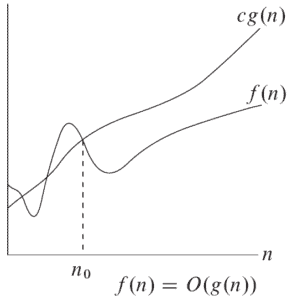## 1. Overview

In this brief tutorial, we’ll learn about how big-O and little-o notations differ. In short, they are both asymptotic notations that specify upper-bounds for functions and running times of algorithms.

However, the difference is that big-O may be asymptotically tight while little-o makes sure that the upper bound isn’t asymptotically tight.

Let’s read on to understand what exactly it means to be asymptotically tight.

## 2. Mathematical Definition

Big-O and little-o notations have very similar definitions, and their difference lies in how strict they are regarding the upper bound they represent.

### 2.1. Big-O

For a given function,is defined as:there exist positive constantsandsuch thatfor all.

Sois a set of functions that are, after, smaller than or equal to. The function’s behavior beforeis unimportant since big-O notation (also little-o notation) analyzes the function for huge numbers. As an example, let’s have a look at the following figure:Here,is only one of the possible functions that belong to. Before,is not always smaller than or equal to, but after, it never goes above.

The equal sign in the definition represents the concept of asymptotical tightness, meaning that whengets very large,andgrow at the same rate. For instance,satisfies the equal sign, hence it is asymptotically tight, whileis not.

For more explanation on this notation, look at an introduction to the theory of big-O notation.

### 2.2. Little-o

Little-o notation is used to denote an upper-bound that is not asymptotically tight. It is formally defined as:for any positive constant, there exists positive constantsuch thatfor all.

Note that in this definition, the set of functionsare strictly smaller than, meaning that little-o notation is a stronger upper bound than big-O notation. In other words, the little-o notation does not allow the functionto have the same growth rate as.

Intuitively, this means that as theapproaches infinity,becomes insignificant compared to. In mathematical terms:In addition, the inequality in the definition of little-o should hold for any constant, whereas for big-O, it is enough to find somethat satisfies the inequality.

If we drew an analogy between asymptotic comparison ofandand the comparison of real numbersand, we would havewhile.

## 3. Examples

Let’s have a look at some examples to make things clearer.

For, we have:

•but•and•andIn general, for, we will have:

•but•and•andFor more examples of big-O notation, see practical Java examples of the big-O notation.

## 4. Conclusion

In this article, we learned the difference between big-O and little-o notations and noted that little-o notation excludes the asymptotically tight functions from the set of big-O functions.